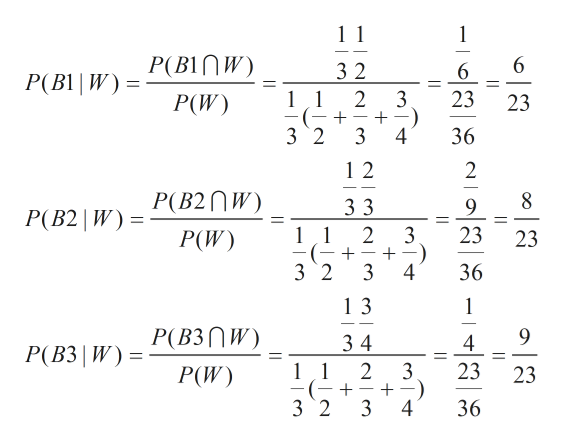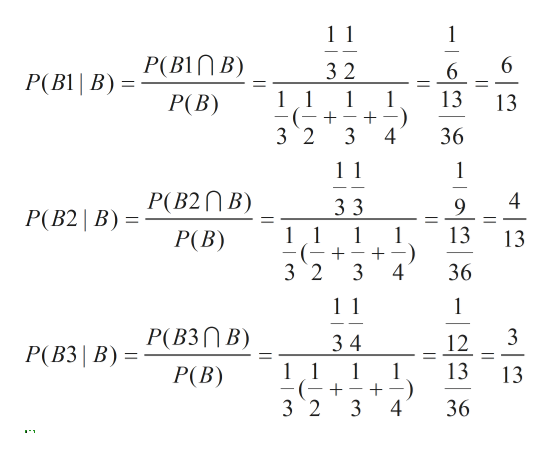# I need help with this problemProblem 9. Consider a game in which balls are divided among three boxes. First, I choose a box from among the three boxes, and then I choose a ball at random from that particular box. Box 1 contains 1 white and 1 black ball; Box 2 contains 2 white and 1 black ball; Box 3 contains 3 white and 1 black ball. After you see what color ball I pick, you guess which Box I chose from, and the game is played over and over. Your objective is to guess the box correctly as often as possible.a) Suppose you know that I pick a box at random with probability (1/3, 1/3, 1/3). Your strategy is to guess the box with highest posterior probability given the observed color. What fraction of the time are you likely to be correct?b) Can you do better given any other strategy?c) Suppose you use the guessing strategy from (a), but I was in fact randomizing with probabilities (1/2, 1/4, 1/4) instead. Now how would your strategy perform?d) Suppose you knew I was randomizing with either set of prior probabilities (but the same one every time). How would you guess which one, and what would you do?

Question
1 views

I need help with this problem

Problem 9. Consider a game in which balls are divided among three boxes. First, I choose a box from among the three boxes, and then I choose a ball at random from that particular box. Box 1 contains 1 white and 1 black ball; Box 2 contains 2 white and 1 black ball; Box 3 contains 3 white and 1 black ball. After you see what color ball I pick, you guess which Box I chose from, and the game is played over and over. Your objective is to guess the box correctly as often as possible.
a) Suppose you know that I pick a box at random with probability (1/3, 1/3, 1/3). Your strategy is to guess the box with highest posterior probability given the observed color. What fraction of the time are you likely to be correct?
b) Can you do better given any other strategy?
c) Suppose you use the guessing strategy from (a), but I was in fact randomizing with probabilities (1/2, 1/4, 1/4) instead. Now how would your strategy perform?
d) Suppose you knew I was randomizing with either set of prior probabilities (but the same one every time). How would you guess which one, and what would you do?

check_circle

Step 1

Hi, we are required to answer the first 3 subparts as per guidelines.

Let the event that box 1 is chosen to draw the ball be represented by B1

Let the event that box 2 is chosen to draw the ball be represented by B2

Let the event that box 3 is chosen to draw the ball be represented by B3

Let the event that the ball is white be represented by W

Let the event that the ball is black be represented by B

If a ball that is chosen is white, it could have come from any of the 3 boxes as all 3 have atleast 1 white ball each. So we must now find the probability that the ball comes from box 1 given the ball is white and then probability that the ball comes from box 2 given the ball is white and then the probability that the ball comes from box 3 given the ball is white. Then we compare all 3 probabilities and the box with highest probability is what we guess when we see that the ball is white. This improves our chances of being correct.

We can do the same thing for black ball ie find out the box that improves our chances of being correct.

From the alculations  shown below, we can see that it's best to guess Box 3 every time a white ball is shown.help_outlineImage Transcriptionclose1 1 1 B1 |W) = P(BINW) P(W) 3 2 6 2 3 23 11 23 (- + _ 3 2 3 4 36 1 2 2 P(B2W) 3 3 9 P(B2 | W) 2 3 23 11 23 P(W) 3 2 3 4 36 1 3 1 P(B3nW) 9 34 1 1 4 P(B3 W) 2 3 23 P(W) 23 + 3 2 3 4 36 fullscreen
Step 2

Similarly, when black ball is shown it's best to guess box 1 to improve chances of being correct.help_outlineImage Transcriptionclose1 1 (B1| B) P(B1nB) P(B) 6 3 2 6 Р(B1| В) 13 11 1 13 ( + 3 2 36 3 1 1 P(B2nB) 4 3 3 9 P(B2 B) 13 11 1 P(B) 13 3 2 36 3 1 11 Р (В3П В) 34 12 Р(В3 | В) 11 1 13 3 Р(В) 3 2 3 4 36 fullscreen
Step 3

Now we we know that we have to guess box 1 when we see a black ball and guess box 3 when we see a white ball to improve chances of being correct. We'll be correct when we guess box 1 and the black ball actully comes from box 1. Black ball may come from either of the 3 ...

### Want to see the full answer?

See Solution

#### Want to see this answer and more?

Solutions are written by subject experts who are available 24/7. Questions are typically answered within 1 hour.*

See Solution
*Response times may vary by subject and question.
Tagged in

### Statistics